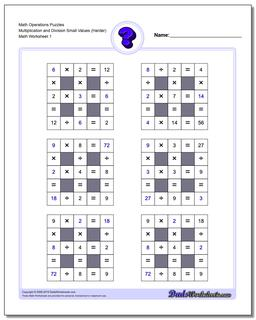# Math Worksheets: Number Grid Puzzles: Number Grid Puzzles: Math Operations Puzzles Multiplication and Division Small Values (Harder)## Math Operations Puzzles Multiplication and Division Small Values (Harder)

PropertyValue
DescriptionMath Operations Puzzles Multiplication and Division Small Values (Harder): Math puzzle worksheets that require students to fill in missing numbers with values up Small Numbers.
Resource TypeWorksheet